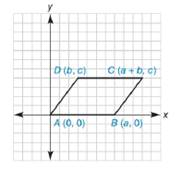Chapter 10.2, Problem 40E### Elementary Geometry for College St...

6th Edition
Daniel C. Alexander + 1 other
ISBN: 9781285195698

#### Solutions

Chapter
Section### Elementary Geometry for College St...

6th Edition
Daniel C. Alexander + 1 other
ISBN: 9781285195698
Textbook Problem
15 views

# Show that quadrilateral A B C D is a parallelogram.To determine

To prove:

The quadrilateral ABCD is a parallelogram.Explanation

To prove that the quadrilateral is a parallelogram, opposite sides are need to be parallel.

By theorem,

If two lines are parallel, then their slopes are equal.

(i.e) If l1l2, then m1=m2.

The slope of the line that contains the points x1,y1 and x2,y2 is given by

m=y2-y1x2-x1 for x2x1

Let mAB- and mDC- are the slopes of the line AB- and DC- respectively.

The given points are A0, 0, and Ba, 0.

Using the slope formula and choosing x1=0, x2=a, y1=0, and y2=0.

mAB-=0-0a-0

mAB-=0a

mAB-=0

The given points are Db, c and Ca+b, c.

Using the slope formula and choosing x1=b, x2=a+b, y1=c, and y2=c.

mDC-=c-ca+b-b

mDC-=0a

mDC-=0

Here mAB-=mDC-

Thus, AB DC

### Still sussing out bartleby?

Check out a sample textbook solution.

See a sample solution

#### The Solution to Your Study Problems

Bartleby provides explanations to thousands of textbook problems written by our experts, many with advanced degrees!

Get Started

#### Find more solutions based on key concepts